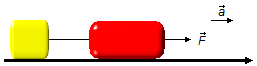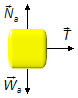Solved Problem on DynamicsPortuguêsEnglish

Two blocks, of masses mA = 0,35 kg and mB = 1,15 kg, are on a frictionless horizontal surface, the blocks are connected by a rope with negligible mass. A horizontal force of constant magnitude equal to 15 N is applied by pulling the two blocks. Find the magnitude acceleration acquired by the system and the tension in the rope connecting the blocks.Problem data
• mass of body A:    mA = 0.35 kg;
• mass of body B:    mB = 1.15 kg;
• force applied to the system:   F = 15 N.

Problem diagram

We use a frame of reference system oriented to the right in the same direction as the force (F) applied, this produces an acceleration (a) in the set.figure 1
Solution

Drawing a free-body diagram for each body, we have for the body A
Horizontal direction
• $$\vec{{T}}$$: force applied to the rope.
Vertical direction
• $${\vec{{N}}}_{\text{A}}$$: normal force of the surface on the body;
• $${\vec{{W}}}_{\text{A}}$$: weight of body A.figure 2
In the vertical direction, there is no motion, normal force and weight cancel out.
In the horizontal direction applying Newton's Second Law
$\bbox[#99CCFF,10px] {\vec{F}=m\vec{a}}$
$T=m_{\text{A}}a \tag{I}$
For body B, we have

Horizontal direction
• $$\vec{{F}}$$: force applied to the system;
• $$-\vec{{T}}$$: force on the rope.
Vertical direction
• $${\vec{{N}}}_{\text{B}}$$: normal force of the surface on the body;
• $${\vec{{W}}}_{\text{B}}$$: weight of body B.figure 3
In the vertical direction, there is no motion, normal force and weight cancel out.
In the horizontal direction applying Newton's Second Law
$F-T=m_{\text{B}}a \tag{II}$
Expressions (I) and (II) can be written as a system of linear equations with two variables (T and a)
$\left\{ \begin{array}{rr} T&=m_{\text{A}}a\\ F-T&=m_{\text{B}}a \end{array} \right.$
substituting (I) into (II) we have the acceleration
$F-m_{\text{A}}a=m_{\text{B}}a\\ F=m_{\text{A}}a+m_{\text{B}}a$
factoring the acceleration
$F=a\;(\;m_{\text{A}}+m_{\text{B}}\;)\\ a=\frac{F}{m_{\text{A}}+m_{\text{B}}}\\ a=\frac{15}{0.35+1.15}\\ a=\frac{15}{1.5}$
$\bbox[#FFCCCC,10px] {a=10\;\text{m/s}^{2}}$
Note: The rope connecting the two blocks is ideal, we can consider it inextensible and of negligible mass, the rope only transmit the force from one block to the other block. The two blocks form a set subjected to the same force, both have the same acceleration, the system behaves as if it were a single block of total mass given by the sum of the masses of the two blocks A and B.

Substituting the acceleration found in expression (I) we have the tension on the rope
$T=0.35\times10$
$\bbox[#FFCCCC,10px] {T=3.5\;\text{N}}$
Note: In the same way we could substitute the acceleration in the expression (II) to obtain the tension on the rope, in this case:
$15-T=1.15\times10\Rightarrow 15-T=11.5\Rightarrow T=15-11.5\Rightarrow T=3.5\;\text{N}$